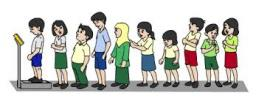# Services 5038

At the camp, half of the boys went on a trip one day, a third went swimming, and 17 boys had various services. How many boys were there in total at the camp? How many boys were on the trip, and how many were swimming?

n =  102
v =  51
k =  34

### Step-by-step explanation:

n/2+n/3+17=n

n = 102

n = 102/1 = 102

n = 102

Our simple equation calculator calculates it.Did you find an error or inaccuracy? Feel free to write us. Thank you!

Tips for related online calculators
Do you have a linear equation or system of equations and looking for its solution? Or do you have a quadratic equation?